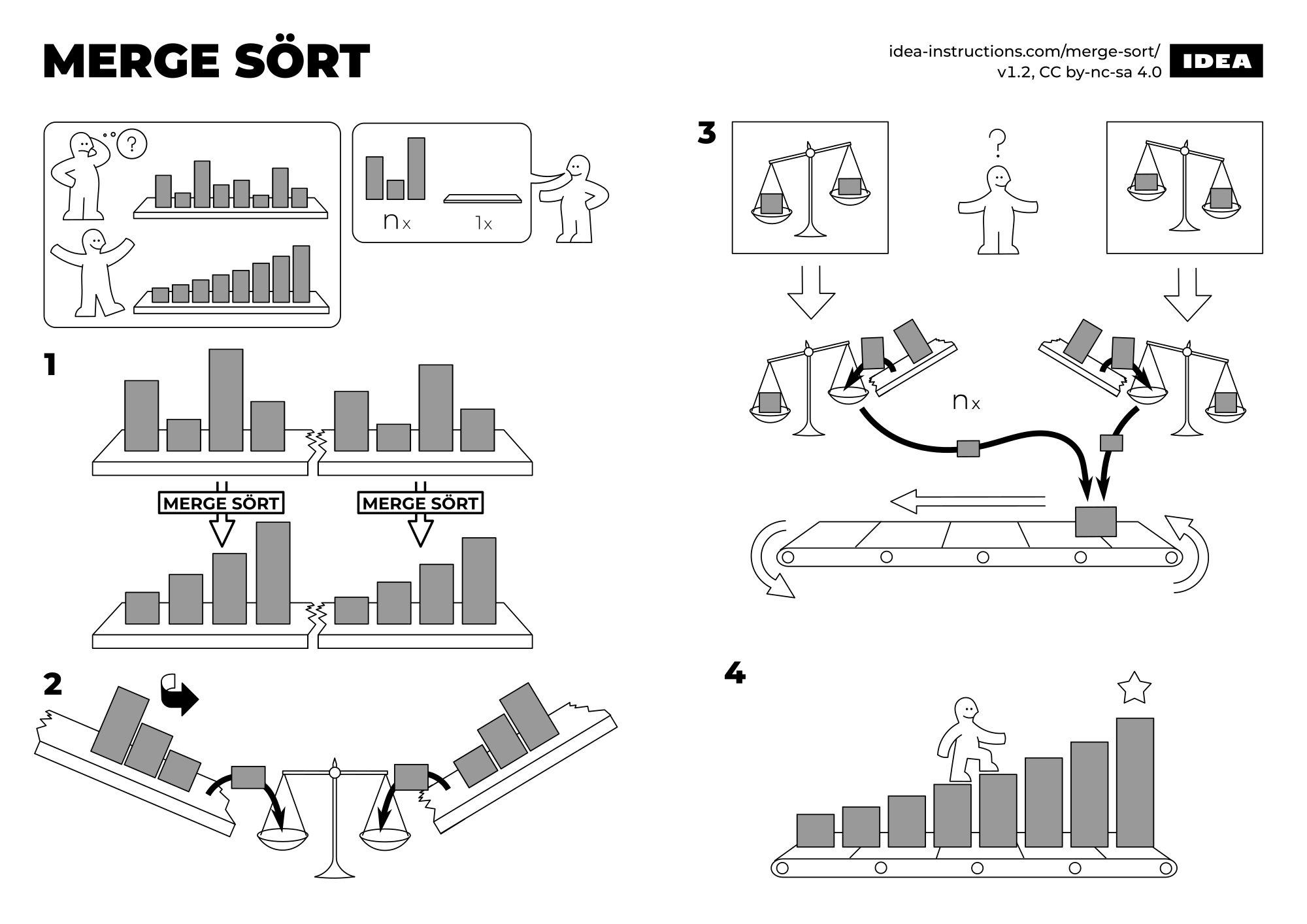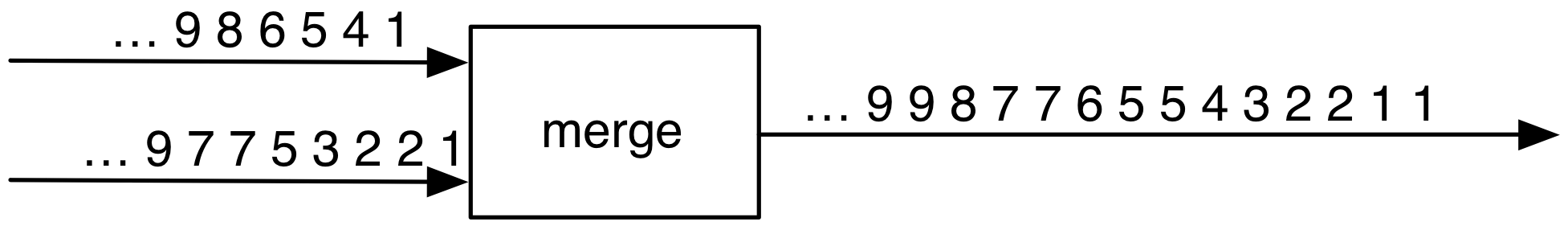« Return to index

# § HOP WPO 5

Assistant: Bjarno Oeyen (bjarno.oeyen@vub.be)

## § Section 1: More Environment Diagrams

### § Exercise 1: A Bug in A Let

Consider the following Scheme program:

There is a bug in this program. Explain why the code does not work. It can help to expand the let expression to lambda.

Draw an environment diagram of executing the expanded code.

## § Section 2: Vectors

So far the only compound data structure that we have seen are cons cells (i.e., pairs). Another compound data structure are vectors: which have a fixed size. They are created using make-vector (or the literal notation vector).

Getting an element of a vector can be accomplished using vector-ref. Modifying of a value stored at a position in a vector (a destructive operation) is possible using vector-set!.

### § Exercise 2: My name is Vector, Scheme Vector

Create a procedure that returns the first non-numeric element stored in a vector.

Hint! Make use of a loop over the index (vectors are indexed by number). You can use vector-length to get the size of a vector.

### § Exercise 3: Map / Filter on vectors

In previous exercise sessions we have seen various implementations of map and filter for lists. Implement vector-map and vector-filter. You must return a new vector: not change the one passed to either vector-map or vector-filter.

Hint! For vector-filter: the new vector can only be created after knowing the size of this vector.

### § Exercise 4: Merge Sort

In this exercise, you have to implement an in-place version of the Merge Sort sorting algorithm.

A quick refresher on the specification of merge sort:Source

Additional resources can be found here: Merge sort - Wikipedia

Hint! During the merge phase (step 3) you will need a temporary vector to avoid overwritten values that you still need during the merge.

The following code can help you get started…

## § Section 3: Streams

Download streams.rkt. Load this file in R5RS using (load "streams.rkt").

The following procedures are defined in this file: cons-stream, head, tail, empty-stream?, accumulate, map-stream, streamfilter, stream-for-each, append-streams, enumerate-interval, zip, print-stream, print-inf-stream, make-read-stream, cartesian-product, pairs, flatten, flatten-inf, flatmap, en list->stream. The empty stream (the-empty-stream) is also defined in this file and is available after loading.

### § Exercise 5: Streams Primer

The following is an explicit definition of the infinite streams of number ones.

• Create a procedure take-n that takes the first n elements of a stream and puts them in a list.
• Create a stream producing all positive integers.
• Create a stream producing all odd positive integers.
• Create a stream of all integers that are not divisble by 2, 3 or 5 (using streamfilter).

### § Exercise 6: Sinus Approximation

For this exercise you have to implement a procedure that approximates a sinus of a number using streams.

$sin(x) = \frac{x}{1!} - \frac{x^3}{3!} + \frac{x^5}{5!} - \frac{x^7}{7!} + ...$

1. Write a procedure that generates an infinite stream of partial sums (see above).
2. Then write a procedure that approximulates the sinus by taking $$n$$ partial sums and calculating the sum.

### § Exercise 7: Collatz Streams

Remember the Collatz conjecture from the first exercise session? The function that is used to generate the next number in the sequence is shown below.

$f(n) = \begin{cases} n / 2 \ &\text{if } n \ \text{is even}\\ 3 \times n + 1 & \text{if } n \ \text{is odd}\end{cases}$

The conjecture is that for every given integer, this sequence goes to the number 1.

1. Create a procedure which creates a stream of Collatz streams.
2. What happens if you make your stream infinite? What happens when this stream is created?

### § Exercise 8: Streams Advanced

In exercise 5, you have implemented streams by making use of an aid procedure. It was also possible to write a definition of ones as follows.

This is called an implicit definition.

1. Write an implicit definition of all integers.
2. Write an implicit definition of fibonacci
3. Write an implicit definition of factorial numbers.

## § Bonus Exercises

### § Bonus Exercise 1: Merge on Streams

Consider two sorted (infinite) streams. Write a procedure that creates a sorted procedure of the two streams, but merged.1. Fixed size, as in after a vector has been created, its size can not be modified. However, upon creation they can have an arbitrary size. ↩︎